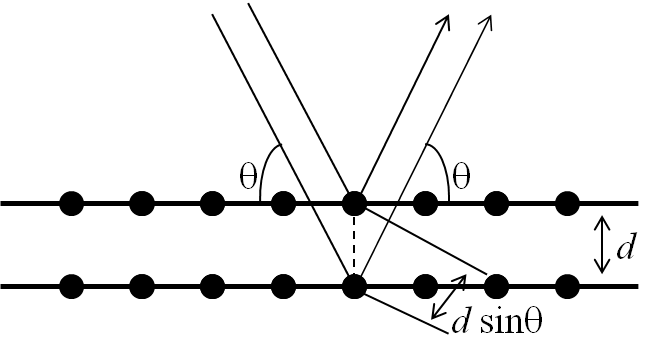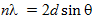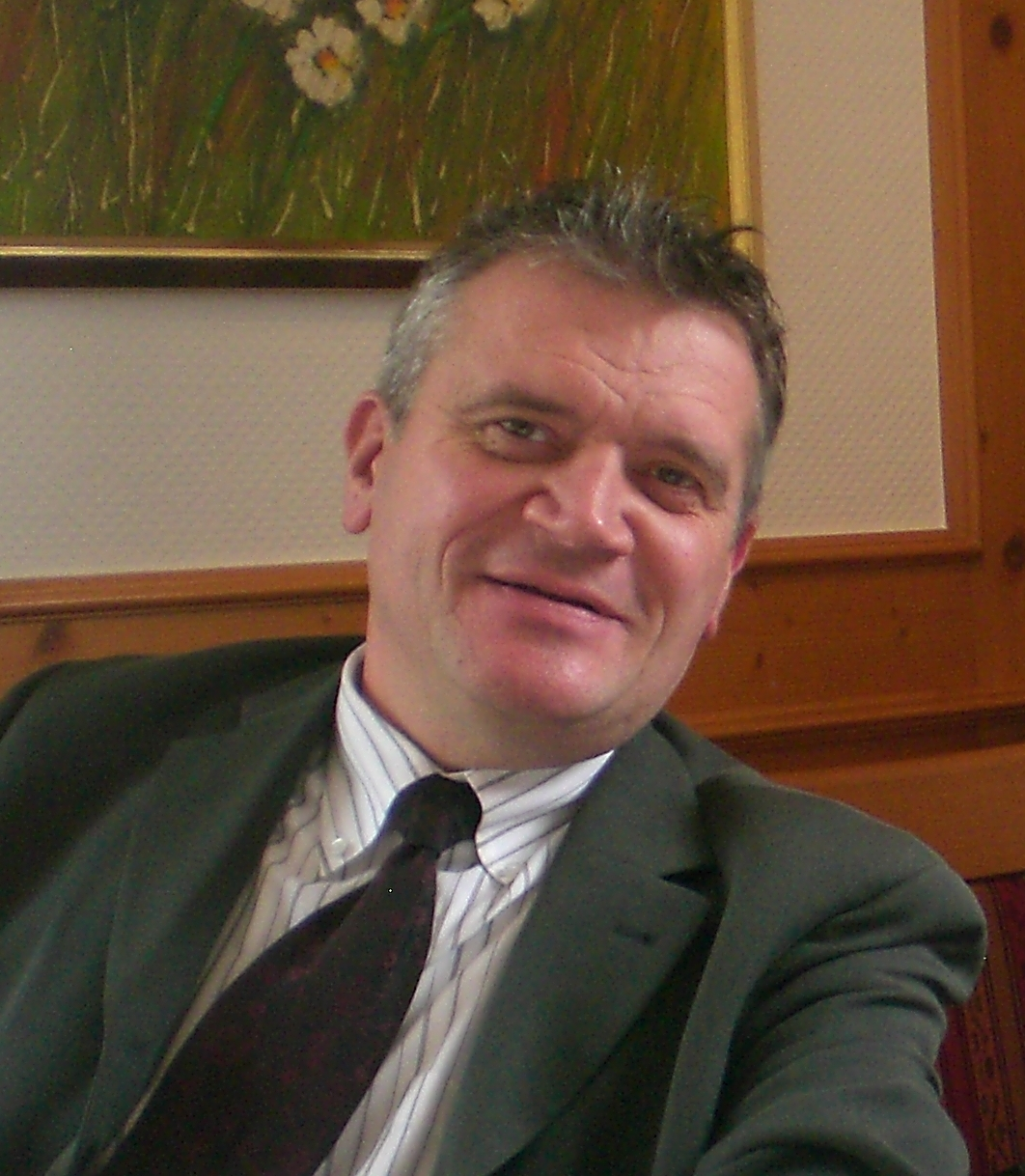# X-Ray Diffractometer (XRD)

Model STOE ID3003 with detector DECTRIS MYTHEN 1K, STOE & CIE GmbH

Device for investigating structural properties

Powder X-ray diffraction allows a non-destructive analysis of multi-component mixtures. It uses X-rays on powder or microcrystalline samples for structural characterization of materials.
For powdered samples the distribution of crystallite orientations will be nearly continuous. Diffracted rays go out from individual crystallites that happen to be oriented with planes making an incident angle θ with the beam satisfying the Bragg equation (see Figure 1). As the crystal axes of the individual grains are randomly oriented, the diffraction pattern produced by such a powder is what one would produce by combining the diffraction patterns for all possible orientations of a single crystal [1,2].
In order to obtain a diffraction pattern from the stimulation of the electrons surrounding the lattice atoms, one needs constructive interference of the reflected X-rays. This only occurs, when the Bragg condition is fulfilled (Figure 1).Fig. 1 Schematic picture of the Bragg diffraction. Two monochromatic beams approach a crystalline solid and are scattered off two different atoms. The path difference between the incident and the reflected rays is 2d sinθ.

The incoming waves are scattered from lattice planes separated by the distance d. When the scattered waves interfere constructively, they remain in phase since the path length of each wave is equal to an integer multiple of the wavelength. The path difference between two waves undergoing constructive interference is given by 2d sinθ, where θ is the scattering angle. This leads to Bragg´s equation:where n is an integer, λ the wavelength of the incident wave, d the spacing between the planes in the atomic lattice and θ the angle between the incident ray and the scattering planes.

  Solid State Physics, N. W. Ashcroft, N. D. Mermin, Cornell University, Thomson Learning, 1976,Chapter 6.  Introduction to Solid State Physics 5th edition, C. Kittel, John Wiley and Sons, 1976, Chapter 2.Prof. Dr.

Professor